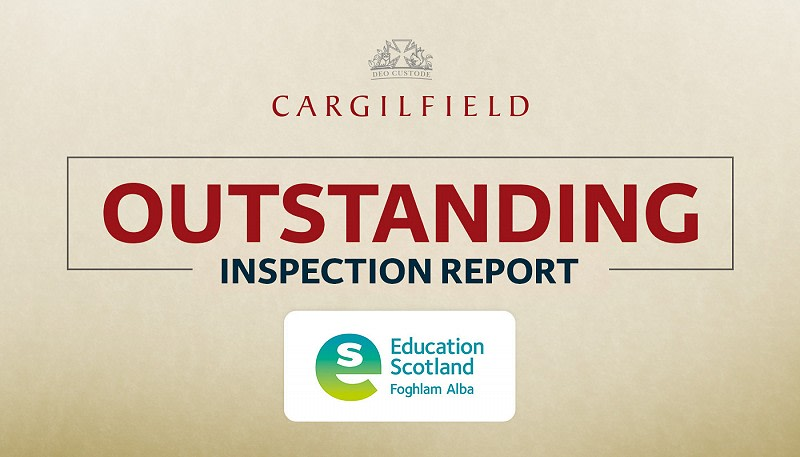• Nursery
• Pre Prep
• Upper School

# Arithmetic techniques and time saving tricks

## Making Maths Easier!

Arithmetic techniques and time saving tricks

Working quickly on paper and being able to carry out calculations in one’s head are useful skills.

The ISEB Mathematics Common Entrance examinations from Autumn 2022 are changing their aural mental arithmetic examination to a quick-fire written exam where pupils are tested on 40 short arithmetic questions in 10 minutes.

Some helpful time saving tricks are listed below. These are types of questions set at CE level but also taught lower down the school with appropriate numbers for the age group.

Double and halving

Example 1)

Twenty-four books are bought at a cost of £3.50 each. What is the total cost?

Working

24 × 3.50

÷ 2 × 2

= 12 × 7

= £84

Example 2)

Sixteen lollies are bought at a cost of £2.25 each. What is the total cost?

Working

16 × 2.25

÷ 2 × 2

= 8 × 4.50

÷ 2 × 2

= 4 × 9

= £36

Further examples

68 × 5 = 34 × 10 = 340 16 × 15 = 8 × 30 = 240 52 × 25 = 26 × 50 = 13 × 100 = 1300

Multiplying by 9, 19, 21, 29 etc.

19 footballs are bought at a cost of £25 each. What is the total cost?

Working

19 × £25 (19 lots of 25)

= 20 × 25 – 1 × 25 (20 lots of 25 subtract 1 lot of 25)

= 500 - 25

= £475

Using Approximations

Example 1)

12 T-shirts are bought at a cost of £7.99 each. What is the total cost?

Working

12 × £7.99

= 12 × £8 – 12 × 1p

= £96 – 12p

= £95.88

Example 2)

A TV sports programme starts at 14 : 35 and last for 2 hours and 55 minutes.

When does it end?

Working

Add 3 hours then jump back 5 mins. 17 : 30

Re-arranging products

Example 1)

2 × 7 × 11 × 5

= 2 × 5 × 7 × 11

= 10 × 77

= 770

Example 2)

25 × 6 × 5 × 4 × 3 × 2

= 25 × 4 × 6 × 3 × 5 × 2

= 100 × 18 × 10 = 18 000

Factorising numbers

Example 1)

437 × 99 + 437

= 437 × (99 + 1)

= 437 × 100

= 43700

Example 2) Extension

34 × 42 + 68 × 29

= 34 × (42 + 2 × 29)

= 34 × (42 + 58)

= 34 × 100

= 3400

R. Farnan

24/11/2021

Posted on

## Cargilfield receives outstanding Inspection Report by Education Scotland!